Mathematics
Easy

Question

# What is the equation of this graph?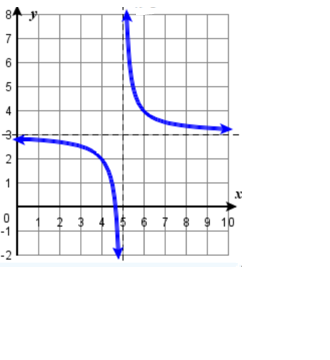## f(x) =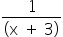+ 5f(x) =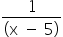+ 3f(x) =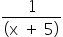- 3f(x) =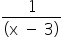– 5Hint:

## The correct answer is: f(x) =+ 3

### Here, we have to find the equation of the given graph.Here, f(x) =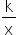has shifted 3 units up and 5 units to the right. 3 units up means: output (f(x)) increased by 3 units 5 units to the right: input (x) decreased by 5 units So, f(x) =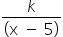+ 3 As we can see, the graph passes through (4, 2). 2 =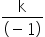+ 3 k = 1 Equation: f(x) =+ 3.Hence, the correct option is D.

There are many ways to transform a graph such as vertical translation, vertical stretch, horizontal translation and horizontal stretch.

### Related Questions to study#### With Turito Foundation.#### Get an Expert Advice From Turito.